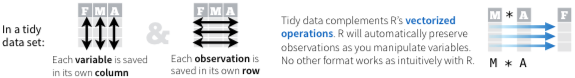This tutorial can be accessed at http://rpubs.com/bradleyboehmke/data_wrangling

## Introduction

### Analytic Process

Analysts tend to follow 4 fundamental processes to turn data into understanding, knowledge & insight:

1. Data manipulation
2. Data visualization
3. Statistical analysis/modeling
4. Deployment of results

This tutorial will focus on data manipulation

### Data Manipulation

It is often said that 80% of data analysis is spent on the process of cleaning and preparing the data. (Dasu and Johnson, 2003)

Well structured data serves two purposes:

1. Makes data suitable for software processing whether that be mathematical functions, visualization, etc.
2. Reveals information and insights

Hadley Wickham’s paper on Tidy Data provides a great explanation behind the concept of “tidy data”### Why Use tidyr & dplyr

• Although many fundamental data processing functions exist in R, they have been a bit convoluted to date and have lacked consistent coding and the ability to easily flow together → leads to difficult-to-read nested functions and/or choppy code.
• R Studio is driving a lot of new packages to collate data management tasks and better integrate them with other analysis activities → led by Hadley Wickham & the R Studio teamGarrett Grolemund, Winston Chang, Yihui Xie among others.
• As a result, a lot of data processing tasks are becoming packaged in more cohesive and consistent ways → leads to:
• More efficient code
• Easier to remember syntax

#### Packages Utilized

``````library(dplyr)
library(tidyr)``````

tidyr and dplyr packages provide fundamental functions for Cleaning, Processing, & Manipulating Data

Go to top

## %>% Operator

Although not required, the tidyr and dplyr packages make use of the pipe operator `%>%` developed by Stefan Milton Bache in the R package magrittr. Although all the functions in tidyr and dplyr can be used without the pipe operator, one of the great conveniences these packages provide is the ability to string multiple functions together by incorporating `%>%`.

This operator will forward a value, or the result of an expression, into the next function call/expression. For instance a function to filter data can be written as:

filter(data, variable == numeric_value)
or
data %>% filter(variable == numeric_value)

Both functions complete the same task and the benefit of using `%>%` is not evident; however, when you desire to perform multiple functions its advantage becomes obvious. For instance, if we want to filter some data, summarize it, and then order the summarized results we would write it out as:

Nested Option:

arrange(
summarize(
filter(data, variable == numeric_value),
Total = sum(variable)
),
desc(Total)
)

or

Multiple Object Option:

a <- filter(data, variable == numeric_value)
b <- summarise(a, Total = sum(variable))
c <- arrange(b, desc(Total))

or

%>% Option:

data %>%
filter(variable == “value”) %>%
summarise(Total = sum(variable)) %>%
arrange(desc(Total))

As your function tasks get longer the `%>%` operator becomes more efficient and makes your code more legible. In addition, although not covered in this tutorial, the `%>%` operator allows you to flow from data manipulation tasks straight into vizualization functions (via ggplot and ggvis) and also into many analytic functions.

To learn more about the `%>%` operator and the magrittr package visit any of the following:

Go to top

## tidyr Operations

There are four fundamental functions of data tidying:

### gather( ) function:

Objective: Reshaping wide format to long format

Description: There are times when our data is considered unstacked and a common attribute of concern is spread out across columns. To reformat the data such that these common attributes are gathered together as a single variable, the `gather()` function will take multiple columns and collapse them into key-value pairs, duplicating all other columns as needed.

Complement to: `spread()`